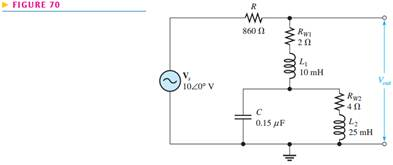Create an Account

Home / Questions / Determine the resonant frequencies and the output voltage at each frequency in Figure 70

Determine the resonant frequencies and the output voltage at each frequency in Figure 70

Determine the resonant frequencies and the output voltage at each frequency in Figure 70.Jul 31 2020 View more View LessSubscribe To Get Solution